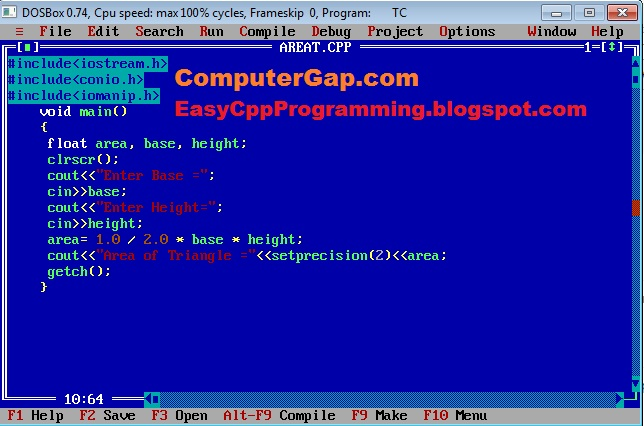# C++ Tutorial With Basic Programs for Beginners

C++ tutorial, C++ programs, C++ code, C++ flowcharts, C++ statements, C++ syntax, C++ examples

## Saturday, March 24, 2018

### Easyway How To Write a Program in C++ for Calculating Area of Triangle When base and height is given

Program Statement: Write a C++ program to input base and height of a triangle and calculates and prints the area of triangle.Input base and height, compute area of triangle c++ program

### Formula for Area of Triangle C++ program when base and height are given

The formula for area of triangle to be used in this C++ program is as as follows:
1. area of triangle = 1 / 2 x base x height

#### How Formula of  Area of Triangle is Transformed into C++ Arithmetic Expressions

In C++ programming, we write the above formula as following C++ expression.

#### area = 1.0/2.0 * base * height

or we can also use the formula: area = 0.5 * base * height

### Source Code for Modern C++ Compilers / IDEs

```#include<iostream>
#include<iomanip>
using namespace std;
int main()
{

float area, base, height;

cout<<"Enter base  = ";
cin>>base;
cout<<"Enter height  = ";
cin>>height;

area = 1.0 / 2.0  * base * height;

cout<<"Area of  Triangle = "<<setprecision(2)<<area<<endl;
return 0;
}
```

### C++ Source Code for Calculating Area of Triangle When Base and Height are Given (for Turbo C++ 3.0 compiler / IDE)

#include<iostream.h>
#include<conio.h>
#include<iomanip.h>

void main()
{

float area, base, height;
clrscr();
cout<<"Enter base  = ";
cin>>base;
cout<<"Enter height  = ";
cin>>height;

area = 1.0 / 2.0  * base * height;

cout<<"Area of  Triangle = "<<setprecision(2)<<area<<endl;

getch();
}

### How This Program works : C++ Source Code for Calculating Area of Triangle When Base and Height are Given

Here is a detailed C++ program with simplified documentation included as C++ comments, so that the logic of C++ Program Area of Triangle can be understood by readers and other C++ programmers easily:

#include<iostream.h>   //include for cin, cout
#include<conio.h>     //include for clrscr()
#include<iomanip.h>  //include for setprecision()

void main()
{

float area, base, height;   //declare variables
clrscr();
cout<<"Enter base  = ";
cin>>base;                          //input base
cout<<"Enter height  = ";
cin>>height;      //input height

area = 1.0 / 2.0  * base * height;  //compute area

cout<<"Area of  Triangle = "<<setprecision(2)<<area<<endl; // show result

getch();
}

Output:
Enter Base: 5.2
Enter Height: 10.8
Area of Triangle = 28.08

Share:

#### 1 comment:

1.A Computer Science portal for geeks. It contains well written, well thought and well explained computer science and programming articles, quizzes and practice/competitive programming/company interview Questions.
website: geeksforgeeks.org

We Love To Hear From You!